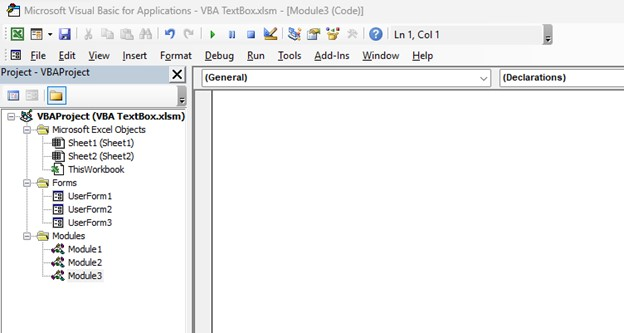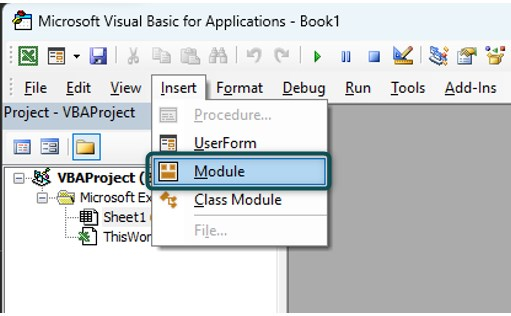# VBA String to IntegerArticle byExcelMojo Team## What is Excel VBA String to Integer?

“VBA String to Integer” refers to converting a string with numerical characters into an integer (whole number) that can be used for mathematical operations or other numeric tasks in excel. The CINT function is commonly used for this purpose.

Let us look at an example. Here, we have a VBA subroutine named “SimpleStringToInteger.” We declare two variables, myString to hold the string “42” and myInteger to store the converted integer. We assign the string value “42” to “myString” and then use the “Cint” function to do a VBA String to Integer convert, which is stored in the “myInteger” variable.

When you run this code, it will print “The converted integer is: 42” in the Immediate window, demonstrating a basic VBA String to Integer conversion.

###### Key Takeaways
1. Always validate that the input string contains valid numeric data before attempting the conversion. Invalid input can lead to errors and unexpected results.
2. Ensure that the variable or expression you pass to VBA String to Integer “Cint” is compatible with integer data types. Attempting to convert incompatible types may lead to errors.
3. Verify that the input string is in a purely numeric format, free from currency symbols, commas, or any non-numeric characters. Cleanse the string, if necessary, before conversion.
4. If you anticipate the possibility of values exceeding the Integer data type’s limits, explore using alternative data types like Long, which offer a broader range for integer values.

### Syntax of CINT Function

The VBA String to Integer “CINT” function has a simple syntax:

CINT(Expression)

Expression: This is the text string you want to convert to an integer.

The CInt function is used for VBA String to Integer conversion. It converts the expressions into the Integer data type in VBA. An “Expression” must be a numerical value falling within the Integer data type’s range in VBA, between -32,768 and 32,767.

### How to use VBA String to Integer?

Here are the steps to use VBA String to Integer:

1. Open VBA Editor: Press ALT + F11 in Excel to open the VBA editor.2. Insert Module: Click “Insert” > “Module” to insert a new module.3. Declare variables for your string and integer. For example:

Dim myString As String

Dim myInteger As Integer

4. Set a string value you want to convert. For example:

myString = “123”

5. The “Cint” function converts the VBA String to an Integer.

myInteger = CInt(myString)

6. You can now use the converted integer in your code.

7. Press F5 or click “Run” > ” to execute your code.

### Examples

#### Example #1 – Basic Conversion

In this example, we will understand the VBA String to Integer conversion process. We’ll begin by assigning a string value to a variable, then proceed to convert this string into an integer using the CInt function.

Finally, we will showcase the usage of the resulting integer value within the code and display it via a message box.

Step 1: In the new module, we first create a subroutine named “BasicConversionExample.”

Step 2: Here, we declare two variables:

• myString as a String type to store the input string.
• myInteger as an Integer type to store the converted integer.

Step 3: Next, we assign the string “123” to the myString variable. It is the input string we want to convert to an integer.

Step 4: Next, we convert the String to an Integer. This line uses the CInt function to convert the myString variable (which contains “123”) to an integer. The result is stored in the myInteger variable.

Step 5: We display a message box using the MsgBox function. It shows the message “The integer value is: ” followed by the value stored in the myInteger variable.

Step 6: Finally, save the code and Run. To execute this code, press F5 or click “Run” in the VBA editor.

Step 7: After running the code, a message box will appear with “The integer value is: 123,” indicating that the string “123” has been successfully converted to an integer.

Here is the full code:

Sub BasicConversionExample()
Dim myString As String
Dim myInteger As Integer
‘ Assign a string value
myString = “123”
‘ Convert the string to an integer
myInteger = CInt(myString)
‘ Use the integer in your code
MsgBox “The integer value is: ” & myInteger
End Sub

#### Example #2 – Handling Errors

In this example, we will see how to assign a string value, attempt to convert it to an integer, and then handle potential conversion errors using error handling in VBA. Depending on the success of the conversion, we will display either an error message or the resulting integer value.

Step 1: In the new module, first, we define a subroutine named “ErrorHandlingExample.”

Step 2: Like example 1, we declare the same two variables.

Step 3: Here, we assign the string “ABC” to the myString variable. This input is not a valid integer.

Step 4: Use the IsNumeric function to check if myString contains a numeric value. This function returns True if the string can be interpreted as a number and False otherwise.

Step 5: If the input string is numeric (the condition in Step 4 is True), we convert it to an integer using the CInt function.

Step 6: If the conversion is successful, we display a message box with the message, “The integer value is: ” followed by the converted integer.

Step 7: If the input string is not numeric (the condition in Step 4 is False), we display an error message using the MsgBox function. The error message indicates that the conversion failed because the input is not a valid number.

Step 8: Save the macro and execute the code by pressing F5 or clicking “Run.”

Step 9: After running the code with the input string “ABC,” it will display a message box with the error message “Conversion failed. Input is not a valid number,” indicating that the input is not a valid numeric string.

Here is the full code:

Sub ErrorHandlingExample()
Dim myString As String
Dim myInteger As Integer
‘ Assign a string value
myString = “ABC”
‘ Attempt to convert the string to an integer
On Error Resume Next ‘ Ignore errors
myInteger = CInt(myString)
On Error GoTo 0 ‘ Reset error handling
‘ Check for errors
If Err.Number <> 0 Then
MsgBox “Conversion failed. Error: ” & Err.Description
Else
MsgBox “The integer value is: ” & myIntegers
End If
End Sub

#### Example #3 – Using InputBox

In this example, we will demonstrate how to prompt the user for input using InputBox in VBA, attempt to convert that input to an integer, and then display either the resulting integer or an error message using error handling.

Step 1: We define a new subroutine named “InputBoxExample” in the new module.

Step 2: Now, we declare the same variables as in the previous examples.

Step 3: We use the InputBox function to prompt the user to enter a number. The input is stored in the myString variable.

Step 4: Then, we attempt to convert the input to an integer with error handling.

Step 5: We check for errors and display the result in this step.

Step 6: Save the macro and run the code. Once you run the code, it will provide a window to enter a number.

Step 7: Once you enter the number and click on OK, the code will display a message box in VBA with either the converted integer value or a message with an integer value of “0” if the input is text.

Note: VBA’s CInt function treats non-numeric input as 0 and does not trigger the error handling mechanism you have in place.

Here is the full code:

Sub InputBoxExample()
Dim myString As String
Dim myInteger As Integer
‘ Prompt the user for input
myString = InputBox(“Enter a number:”)
‘ Attempt to convert the input to an integer
On Error Resume Next ‘ Ignore errors
myInteger = CInt(myString)
On Error GoTo 0 ‘ Reset error handling
‘ Check for errors
If Err.Number <> 0 Then
MsgBox “Conversion failed. Error: ” & Err.Description
Else
MsgBox “The integer value is: ” & myInteger
End If
End Sub

### Important Things To Note

1. Ensure that the input string you intend to convert to an integer is a valid numeric value. The CInt function cannot handle non-numeric characters or empty strings, and attempting to convert them will result in errors.
2. Be aware of the limitations of the Integer data type in VBA, which ranges from -32,768 to 32,767. Attempting to convert a VBA string to integer with a value outside this range will cause an “Overflow” error.
3. Implement error handling in your code when using CInt. If the input string cannot be converted to an integer, it’s advisable to use techniques like “On Error Resume Next” and “On Error GoTo” to handle errors and prevent code crashes.
4. Verify that the input string uses a numeric format without extra characters like currency symbols or commas. Remove any non-numeric characters before attempting the conversion.
5. We use VBA String to Integer Array to create and manage arrays for storing integer values obtained through string conversions, considering dimensioning, validation, and error handling.

1. How to VBA Convert Integer to String with Leading Zeros?

You can use the Format function to convert an integer to a string with leading zeros. For example, Format(myInteger, “00000”) will convert the integer 42 to the string “00042” with leading zeros.

2. How to Concatenate String and Integer in Excel VBA?

To concatenate a string and an integer in Excel VBA, use the & operator. For instance, “Value: ” & myInteger will concatenate the string “Value: ” with the integer value stored in the variable myInteger.

3. Why is VBA String to Integer Not Working?

VBA String to Integer conversion may not work for various reasons. Here are some possible reasons:

· Invalid Input: If the input string contains non-numeric characters or is empty, the CInt function, used for conversion, will fail.

· Overflow: An overflow error will occur if the integer value is too large to fit within the Integer data type’s range (from -32,768 to 32,767). Consider using a different data type, like Long, to accommodate larger numbers in such cases.

· Variable Declaration: Ensure variables are correctly declared with the appropriate data types (e.g., Integer for integers and String for strings).

This has been a guide to VBA String to Integer. Here we explain how to use VBA String to Integer along with examples & downloadable excel template. You can learn more from the following articles –